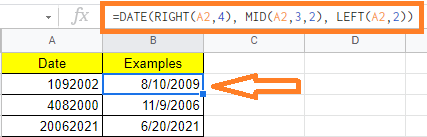# How to Convert Text to Date in Google Sheets? (With Examples)

In Google Sheets, we have n number of options to represents dates. However, in a few instances, the Dates will be understood as text when we are importing files to Google Sheets.  And thus if we want to perform some operations with Dates in Google Sheets, it will lead to improper results or errors. To overcome this issue, we will have to convert text to date. Let’s understand how to convert text to date with the help of Google Sheet tips provided on this page

## How to Find Out If a Date Is Actually a Text Value in Google Sheets?

Using the ISDATE function, you may determine whether a value in a cell is a date or not.

If the value in a cell is a date, then the function returns TRUE otherwise it returns FALSE in Google Sheets.

The valid date will be as follows, we need to specify the day followed by the month and then a year.

Below are other ways to tell if the date is actually a text value,

• If the contents of the cell are aligned to the left, then it is not date but the text value
• If the contents of the cell are aligned to the right, then it is a date.
• In some cases, when you click on the cell, you will see a leading apostrophe to the date in the formula bar, signaling that it is a text value

Example: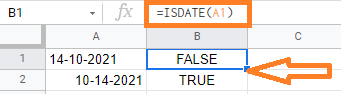In the above example,

• If you notice that the A1 cell is left-handed aligned and also the date format is mentioned in date-month-year, thus the result is FALSE
• If you notice that the A2 cell is right-handed aligned and also date format is mentioned in month-date-year, thus the result is TRUE.

## How to Convert Text to Date in Google sheets?

Once you identify the date is considered as a text in google sheets, then you can convert it to date.

Below are the situations where the date is considered as text,

• A previous user may have worked on the sheet and saved the date values as text, either by appending an apostrophe (‘) to the date value or by changing the date to plaintext format.
• Date formats are understood by the Google sheets based on the region and language settings. When the date format is mentioned in dd-mm-yyyy. Note that Google Sheets accept only date format with mm-dd-yyyy.
• If the user gives an invalid date like, 14102021, then it is considered as a text.

Below are some of the methods where you can convert the text as a date value,

### Using DATEVALUE and TO_DATE Function to Convert Text to Date in Google Sheets

To convert the text format to date in google sheets, you could use any of the DATEVALUE and TO_DATE functions.

The DATEVALUE function takes a date value in any format and returns the same date as a serial number.

Syntax:

DATEVALUE(date_string)

In the above syntax,

• date_string represents the date in TEXT format

The TO_DATE function converts a given serial number to its corresponding date.

Syntax:

TO_DATE(value)

In the above syntax,

• the value represents the number that has to be converted to date format

In order to use the TO_DATE function, we need to call the DATEVALUE function in google sheets.

Example: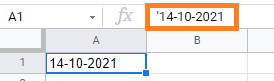In the above example, the date is prefixed with (‘)-apostrophe which means it is considered as TEXT value.

### Using DATE Value Function in Google Sheets

It is important to note that there’s a leading apostrophe, indicating that this is a TEXT value.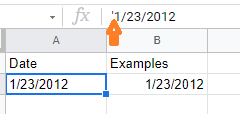To convert this TEXT date to a correct DATE value, use the TO DATE function in combination with the DATEVALUE function, as shown below:

=TO_DATE(DATEVALUE(A2))

The DATEVALUE function will convert the text date to a number value, which will then be converted back to an actual date using the TO DATE function, as shown in the image below: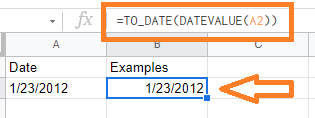### How to Convert Text to Date when Date is in an 8-digit Number Format?

When we import a Google Sheets from a PDF version to a sheet version, the dates will be imported in text format. For example, if your date format is 12/02/2021 in PDF and when you import this version, it will be formatted as 12022021 which is an 8 digit format.

In these cases, we need to convert text to date. Let us understand how to do this with the help of the steps listed below:

• Step 1: Consider the following dataset where we have the Date in 8 digit text format.
• Step 2: Firstly, we need to extract the Year. This is made up of the text date’s last four digits (which are to the right of the value). To achieve this, use the formula “=RIGHT(A2,4)”.
• Step 3: Now we need to extract the Month. This is made up of the text date’s third and fourth digits (which are in the middle of the value). To extract the month, we need to use the formula “=MID(A2,3,2)“.
• Step 4: The last step is to extract the day from the text date format. This is made up of the text date’s first two digits (which are to the left of the value). To extract the date, we need to use the formula “=LEFT(A2,2)“.
• Step 5: Now combine all the formulas listed in Step 2, 3, 4. So our final formula will be “=DATE(RIGHT(A2,4), MID(A2,3,2), LEFT(A2,2))“.
• Step 6: Press the “Enter” button. The results will be as follows.